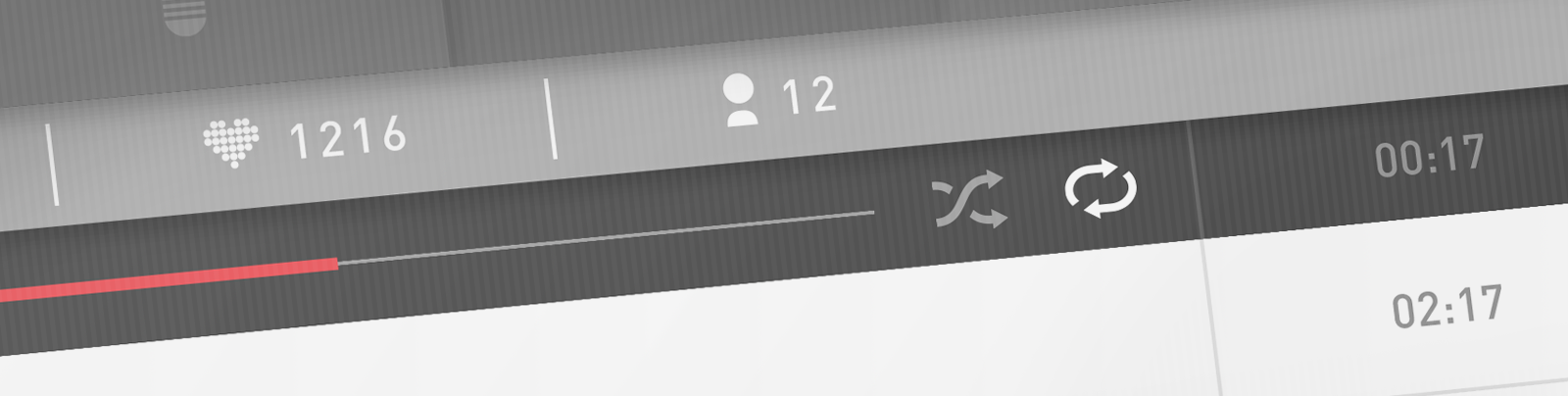We're hiring! Are you a developer who wants to work on interesting technical challenges with a small, passionate team here in London? For more information, visit the Mixlr jobs homepage.

Playlist shuffle was a feature added to the Mixlr desktop app in 2015 after popular demand from users. Shuffling can be approached in different ways from a technical perspective, but is hard to get “right” from a user perspective. Youtube solves the problem by randomly sorting the playlist, which may be fine for a youtube listener playlist, but a Mixlr broadcaster probably wouldn’t want their lists being randomly rearranged. If you can think back to the way Winamp performed with shuffled tracks in a playlist, you may have had an experience where one of your favourite tracks never seemed to play.At Mixlr, we wanted to nail the user experience for shuffling, using a high quality (in terms of bias) algorithm/technique. The shuffle feature was well received, and we would like to share our method for both learning and re-use; please read on for the juicy details.

#### Getting the shuffle right

Writing an algorithm to perform a shuffle, at first thought, sounds quite easy. A quick solution might involve selecting a random number from $$1$$ to $$N$$ (where $$N$$ is the number of tracks you have loaded in your playlist) and choosing that track to play. This process could simply be repeated to select the next track. Sounds OK in principle, but it’s not actually a good solution for several reasons:

• The shuffle is biased, in the worst case it could result in one track being played more than others, whilst another track never gets played.
• Each track has a fixed (and biased!) probability of playback. That means there’s a possibility that one track could be selected multiple times in a row.
• There is no control over the probability - i.e. to manipulate the selection likelihood of a particular track

There are algorithms available for shuffling that solve the biasing issue (e.g. the Fisher-Yates-Knuth shuffle). However, we want more control over the shuffle to subjectively increase the quality of track playback order from a human perspective.

#### The shuffle experience

Shuffling in a way that “feels right” is a challenge in and of itself; having a intuitive user experience would have to inform algorithm design. There were certain requirements:

• Randomness - a proper random selection rather than a playlist sort. Because it feels more authentically random if you hear a track repeated in a short period.
• Controlling repetition - yes we want randomness, but not to the point of annoyance. It should be much less likely (but still possible) to hear the same track repeated in a short period.
• All tracks matter - if we have $$N$$ tracks, and $$N - 1$$ different tracks have played, it should be much more likely to select the track $$T_j$$ which hasn’t played, rather than the previously selected track $$T_{\mu}$$.

These requirements mean that this problem is different to shuffling a pack of cards. We need to assign and compute the probability of each track playing and make a random selection based on all probabilities (i.e. a type of stochastic roulette wheel selection).

#### Shuffling using random sampling

Random sampling is a method that is straightforward to understand and implement. The general idea behind random sampling to select individuals of a population using random numbers; in our case we have a population of tracks. Our approach differs from simple random sampling as we assign a propensity (weighted probability) to each track which is modified when a track is played (set to zero) but slowly increases over time. This form of selection requires us to record the sum of the propensity of all tracks $$P_{total}$$ and multiply it by a random number $$r_1$$ in the range $$[0.0, 1.0]$$ to generate a “target propensity” $$P_{target}$$. Note that we recommend using the Mersenne Twister psuedo-random number generator, it has a period that far exceeds the requirements of this application yet is computationally inexpensive.

$$P_{total} = \sum_{i=1}^NP_i(T)$$
$$T_{\mu} = \min\left\{\mu|\sum_{j=1}^{j=\mu} a_j(x) > r_1P_{total}(x)\right\}$$

Using $$P_{target}$$ we can select an individual track by assessing its “position” in the list of tracks propensities (by summing propensities until we hit the target).

Here are the major stages to our approach:

1. Assign every track a propensity - in our case start the integer $$N$$ (where $$N$$ is total the number of tracks).
2. Pick a random number $$r_1$$ in the range $$[0.0, 1.0]$$ and multiply it by the total track propensities $$P_{total}$$ to get a “target propensity” $$P_{target}$$.
3. Iterate over tracks and sum propensities until $$P_{target}$$ is reached - select the last track $$T_{\mu}$$ we iterated over to play.
4. Reduce the propensity of the last track $$T_{\mu}$$ we played - in our case we set it to $$0$$.
5. Update the propensities of any other tracks that are less than $$N$$ (i.e. ones that have been recently played) - in our case we add $$1$$.
6. Recalculate the total propensity $$P_{total}$$ of all tracks.
7. GOTO 2.

This method satisfies the requirements of our shuffle experience: it provides good quality randomness, limits repetition and increases the likelihood of a previously unheard track getting airtime.